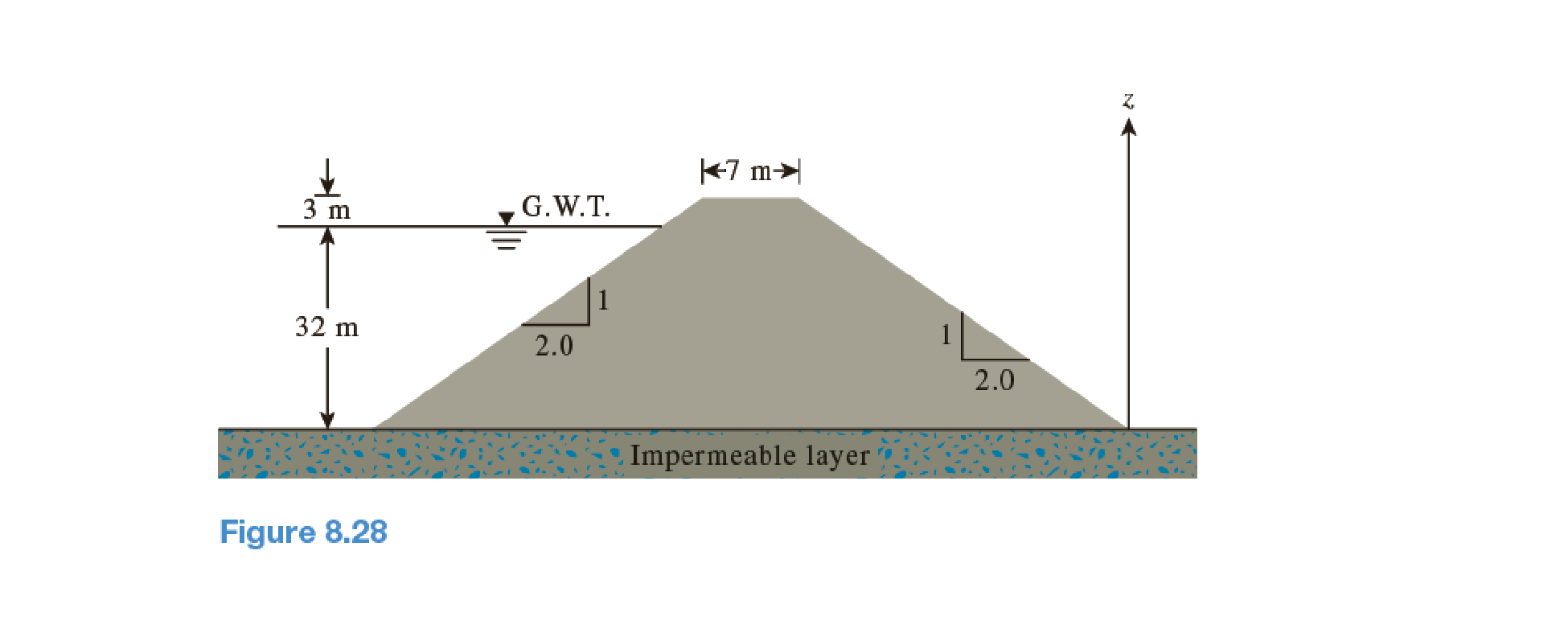Chapter 8, Problem 8.12PPrinciples of Geotechnical Enginee...

9th Edition
Braja M. Das + 1 other
ISBN: 9781305970939

Solutions

Chapter
SectionPrinciples of Geotechnical Enginee...

9th Edition
Braja M. Das + 1 other
ISBN: 9781305970939
Textbook Problem

An earth dam section is shown in Figure 8.28. Determine the rate of seepage through the earth dam using Pavlovsky’s solution. Use k = 4 × 10–5 mm/s.To determine

Find the seepage rate through the earth dam using Pavlovsky’s solution.

Explanation

Given information:

The height of the water level H is 32 m.

The height of the earth dam Hd is 35 m.

The length of earth dam top B is 7 m.

The hydraulic conductivity of the permeable soil layer k is 4×105mm/s.

Calculation:

Determine the value of α using the relation.

tanα=VH

Here, V is the vertical distance in the slope and H is the horizontal distance in the slope.

Substitute 1 for V and 2 for H.

tanα=12α=tan1(0.5)α=26.57°

Determine the distance h2 value using the relation.

h2=Bcotα+Hd(Bcotα+Hd)2h12

Substitute 7 m for B, 26.57° for α, and 35 m for Hd.

h2=7cot26.57°+35(7cot26.57°+35)2h12=7tan26.57°+35(7tan26.57°+35)2h12=38.51,482.3h12 (1)

Determine the value of h2 using the relation.

Hh1cotβlnHdHdh1=h2cotα

Substitute 32 m for H, 26.57° for β, 35 m for Hd, (38.51,482.3h12) for h2, and 26.57° for α.

32h1cot26

Still sussing out bartleby?

Check out a sample textbook solution.

See a sample solution

The Solution to Your Study Problems

Bartleby provides explanations to thousands of textbook problems written by our experts, many with advanced degrees!

Get Started

What function does the compound rest serve?

Precision Machining Technology (MindTap Course List)

Define an attribute, and provide three examples.

Systems Analysis and Design (Shelly Cashman Series) (MindTap Course List)

List products that contain embedded computers. List the disadvantages of in-vehicle technology.

Enhanced Discovering Computers 2017 (Shelly Cashman Series) (MindTap Course List)